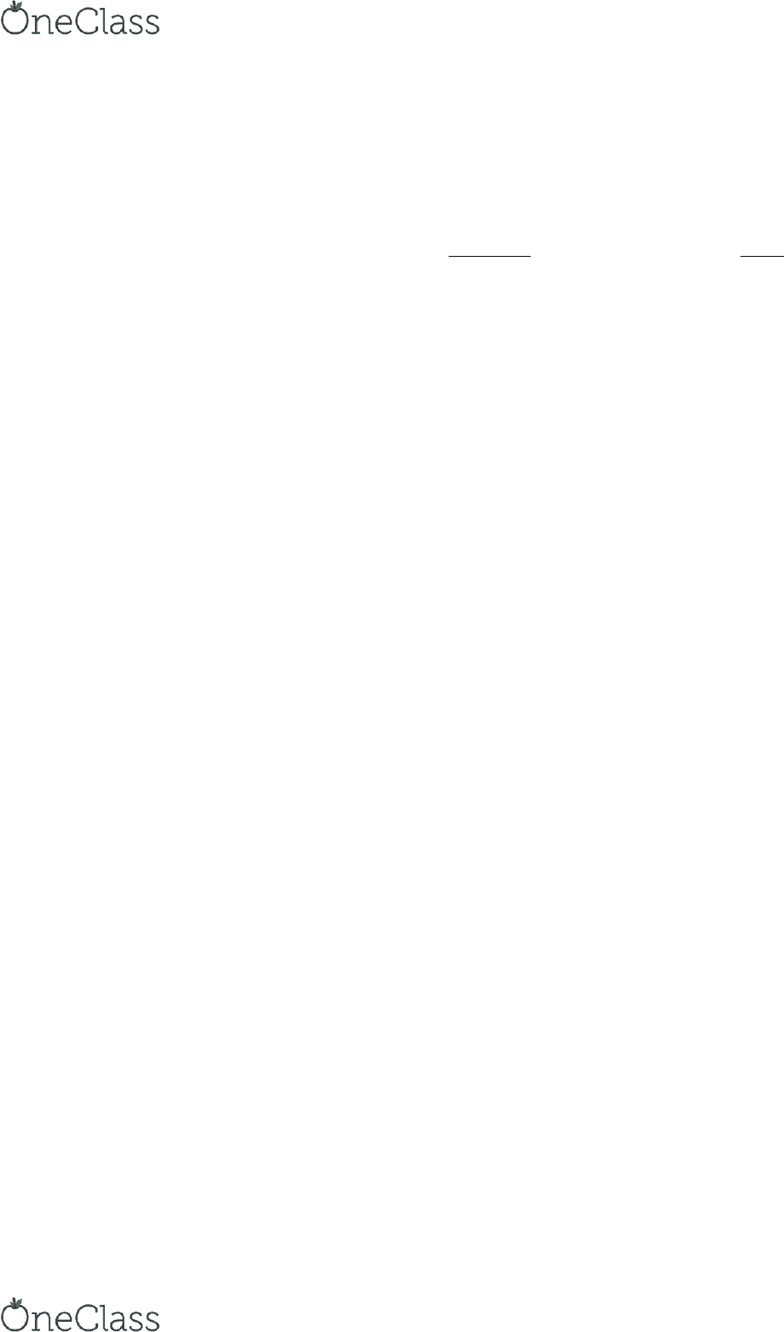Textbook Notes (280,000)
CA (170,000)
McMaster (10,000)
COMMERCE (2,000)
Chapter 6

# COMMERCE 1AA3 Chapter Notes - Chapter 6: Round-Off Error, Weighted Arithmetic Mean

Department
Commerce
Course Code
COMMERCE 1AA3
Professor
Chapter
6

This preview shows page 1. to view the full 4 pages of the document.Chapter 6 In-Class Practice Questions
Question 1
Green Company recorded the following inventory transactions during 2006.
Purchases
Sales
Units
Unit Cost
Units
April
1
Beginning inventory
45
\$8
25
Purchase
150
\$9
May
4
Purchase
65
\$10
16
Sale
120
\$16
June
4
Purchase
50
\$12
20
Sale
80
\$18
Calculate the cost of goods sold and ending inventory assuming the following cost flow
assumptions: (a) FIFO, and (b) weighted average under:
(1) Periodic Inventory System
(2) Perpetual Inventory System
Cost of goods available for sale = 45x8 + 150x9 + 65 x 10 + 50x12 = \$2,960
Units available for sale = 45 + 150 + 65 + 50 = 310
Units sold = 120 + 80 = 200
Units in ending inventory = units available for sale units sold = 310 200 = 110
FIFO and Periodic
Cost of goods sold = 45x8 + 150x9 + 5*10 = \$1,760
Cost of ending inventory = cost of goods available for sale cost of goods sold
= 2,960 1,760 = \$1,200
OR
Cost of ending inventory = 50 x12 + 60x10 = \$1,200
FIFO and Perpetual yields the same results
find more resources at oneclass.com
find more resources at oneclass.com